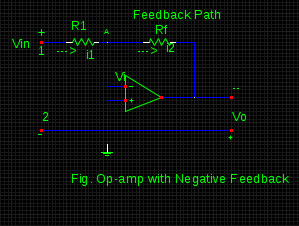# Virtual ground and summing point concept of Op-amp with negative feedback.

## (I) Why do the virtual ground strategy is adopted in case of Op-amps with negative feedback mechanism corresponding to the below figure ?a. Due to reduction in an input voltage at inverting terminal to zero
b. Due to reduction in an input voltage at inverting terminal to unity
c. Due to increase in an input voltage at inverting terminal greater than unity
d. All of the above are correct since condition of operation exists at different phases.

Correct Answer : a. Due to reduction in an input voltage at inverting terminal to zero

Explanation :

The notion of virtual ground originates from the condition when input voltage at the inverting terminal is insisted to reach a very minute value approximately to almost zero value. Thus, the point at which inverting terminal find its connection is significantly at the ground voltage and therefore, it is regarded at the virtual ground level. The reason behind getting reduction of input voltage to zero is the positive potential attained by point A and existence of the output voltage at the same time. Again, some part of of output is fed back to input terminal out of phase. However, the sum of these two voltages become equal to zero.

## (II) According to above figure, 'A' is referred as the summing point because of ______.

a. Addition of input and feedback currents at point 'A'
b. Addition of only input currents at point 'A'
c. Both a & b
d. None

Correct Answer : a. Addition of input and feedback currents at point 'A'

Explanation :

Summing point is basically a point where all input as well as out put or (may be feedback) are summed together. This point simply performs summation of all incoming currents coming towards it. In figure, point 'A' is a summing point. Since the configuration of Op-amp is exhibited to be of negative feedback in above drawn figure, so some part of output is connected at the input through point 'A' is also taken into consideration for the purpose of algebraic sum evaluation.
Generally, the concept of summing point and virtual ground are explained simultaneously for the semantic configuration requirement .